# 不懂送女朋友什么牌子的口红？没关系！Python 数据分析告诉你。JackTian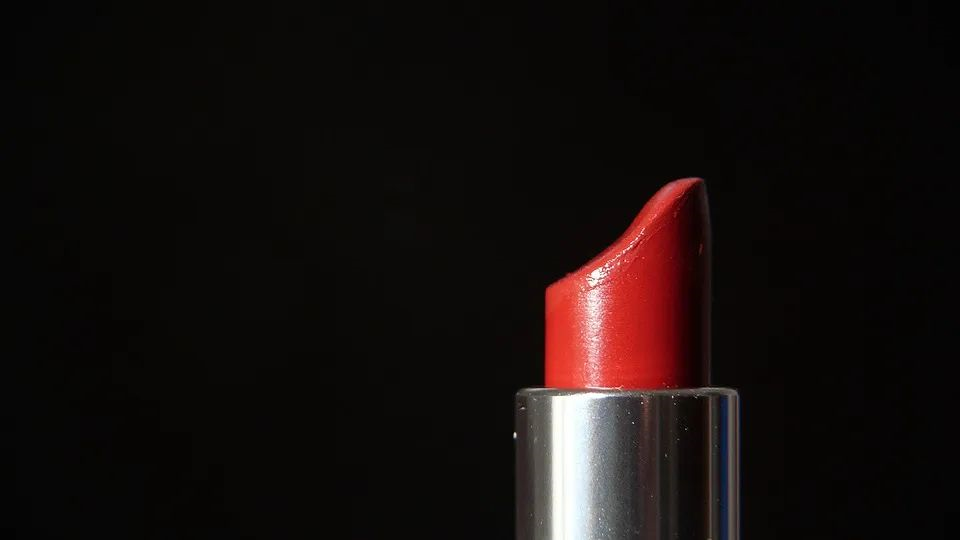### 一、案例说明

#### 1、案例背景

520情人节，不懂送女朋友什么牌子的口红？没关系！Python 数据分析告诉你。

1、哪些价格区间的口红卖的最好？

2、口红销量分布情况。

3、销量前10的口红有哪些？

4、销量前10的店铺。

5、商品价格和销量的关系。

#### 3、数据字段的说明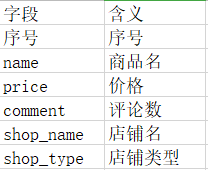#### 4、数据分析的流程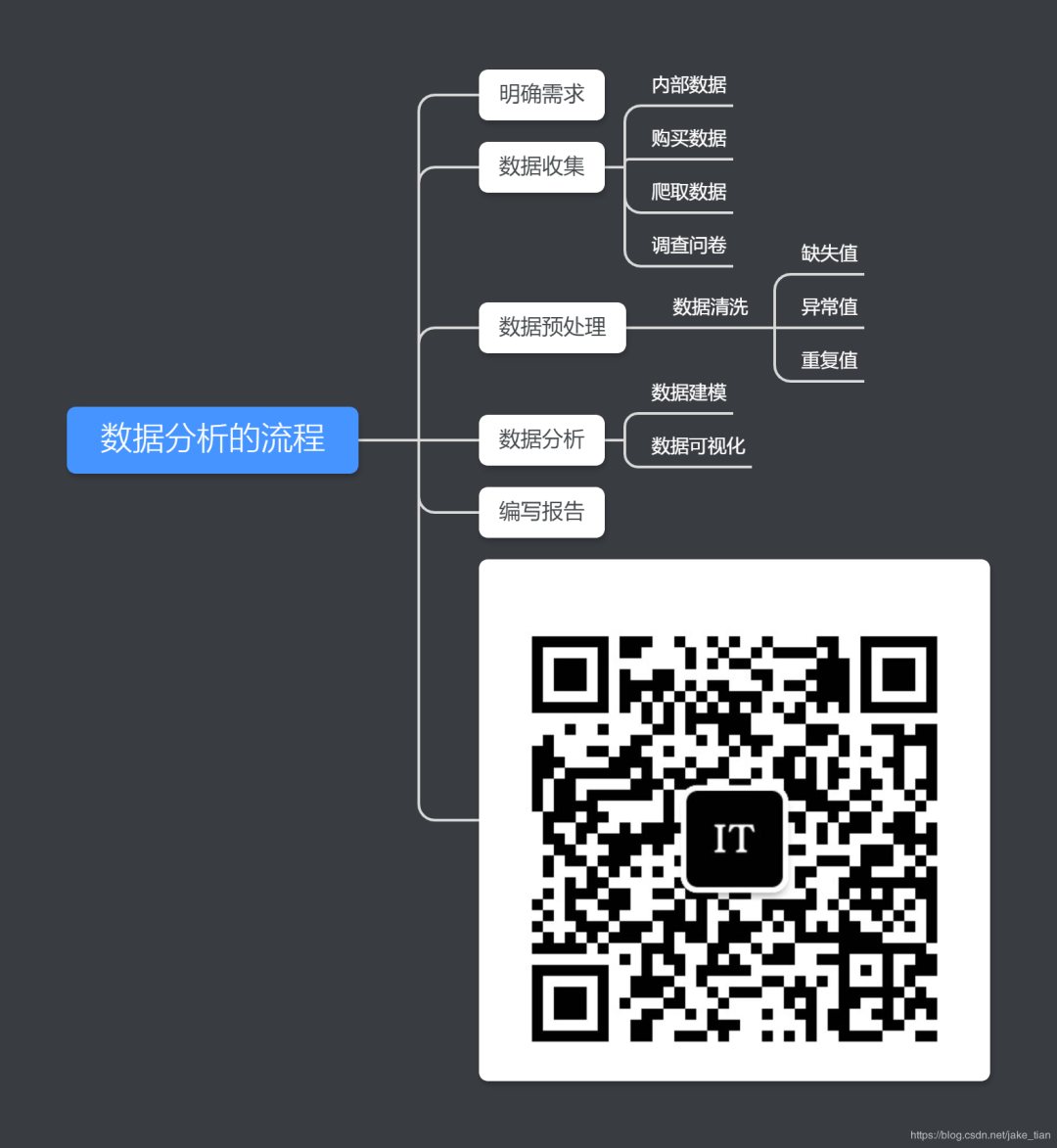### 二、数据预处理

#### 数据清洗

##### 1、首先从csv文件中导入数据

import pandas as pd import matplotlib.pyplot as plt #读取数据dataframe = pd.readcsv('jddata.csv',encoding = 'gb18030')#这里不能使用utf-8print(dataframe.shape)

(3816, 6)

##### 2、缺失值处理
data = dataframe.dropna(how='any')data.head()print(data.shape)

(3610, 6)

# inplace=True表示原地修改数据集data.dropna(axis=0, inplace=True) # 对删除后缺失值后的数据集，再次进行缺失值统计data.isnull().sum(axis=1)

#### 数据转换

##### 1、将评论的+和万字修改
def dealComment(comm_colum):    num = str(comm_colum).split('+')    if '万' in num:        if '.' in num :            num = num.replace('.','').replace('万','000')        else:            num = num.replace('.','').replace('万','0000')    return numdataframe['comment'] = dataframe['comment'].apply(lambda x: dealComment_num(x))#转换成int类型dataframe['comment'] = dataframe.comment.astype('int') data = dataframe.drop('comment',axis = 1)print(data.head(10))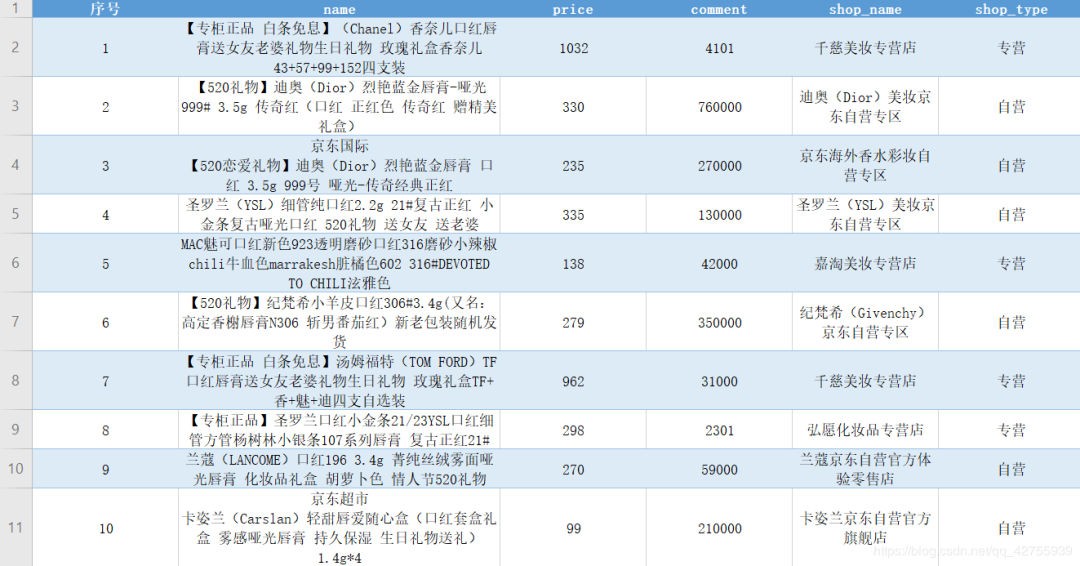### 三、数据分析

#### 1、口红价格分布区间

import pandas as pd import matplotlib.pyplot as plt#读取数据data = pd.read_csv('jd_data.csv',encoding = 'gb18030')plt.rcParams['font.sans-serif']=['SimHei'] #用来正常显示中文标签plt.figure(figsize=(10,8))price = data[data['price'] < 1000]plt.hist(price['price'], bins=10, color='brown')plt.xlabel('价格')plt.ylabel('商品数量')plt.title('价格商品分布')plt.show()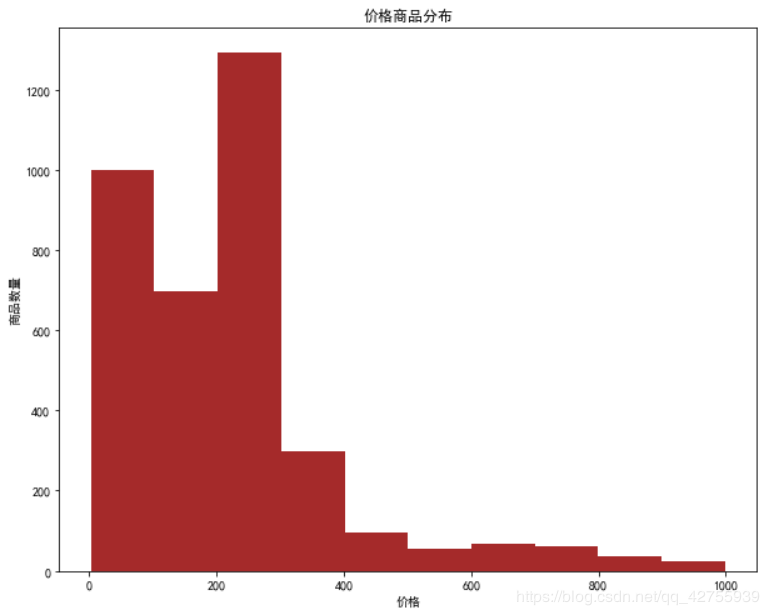1、口红的价格绝大多数在0-500元的区间之内，但是也有口红的售价达到了1000元，哈哈努力挣钱吧。

2、其中200-300元价位的数量非常的高，超过了1200，而且价格超过300元的有明显的减少趋势，哈哈价格才是王道。

#### 2、销量分布情况

#销量分析sale_num = data[data['comment'] > 100]plt.figure(figsize=(10,8))#print(len(sale_num)/len(data))  #查看下大致的区间分布plt.hist(sale_num['comment'], bins=20, color='blue')plt.xlabel('销量')plt.ylabel('数量')plt.title('销量情况')plt.show()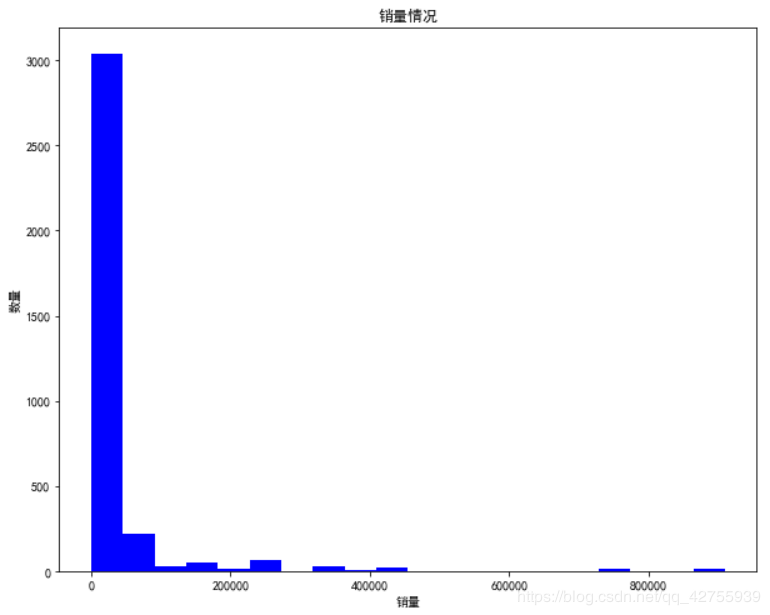1、销售量基本是在20万以内。

2、销售量在10万以内的占了绝大多数

3、还有极个别的店铺销量竟然超过了100万

#### 3、销售前10的口红

#销售前10的口红#抽取商品标题的简略信息def get_title(item):    title = item.split(' ')    return titledata['small_name'] = data['name'].apply(lambda x: get_title(x)) data1 = data.drop('name',axis = 1)top10Lipstick = data1.sort_values('comment',ascending=False)print(top10Lipstick.head(10))title = top10Lipstick['small_name'][:10]sale_num = top10Lipstick['comment'][:10]plt.figure(figsize=(10,8),dpi = 80) plt.bar(range(10),sale_num,width=0.6,color='red')plt.xticks(range(10),title,rotation=45)#plt.ylim((9,9.7))   #设置y轴坐标plt.ylabel('数量') plt.xlabel('标题')  plt.title('销量前10的糖果')for x,y in enumerate(list(sale_num)):       plt.text(x,float(y)+0.01,y,ha='center')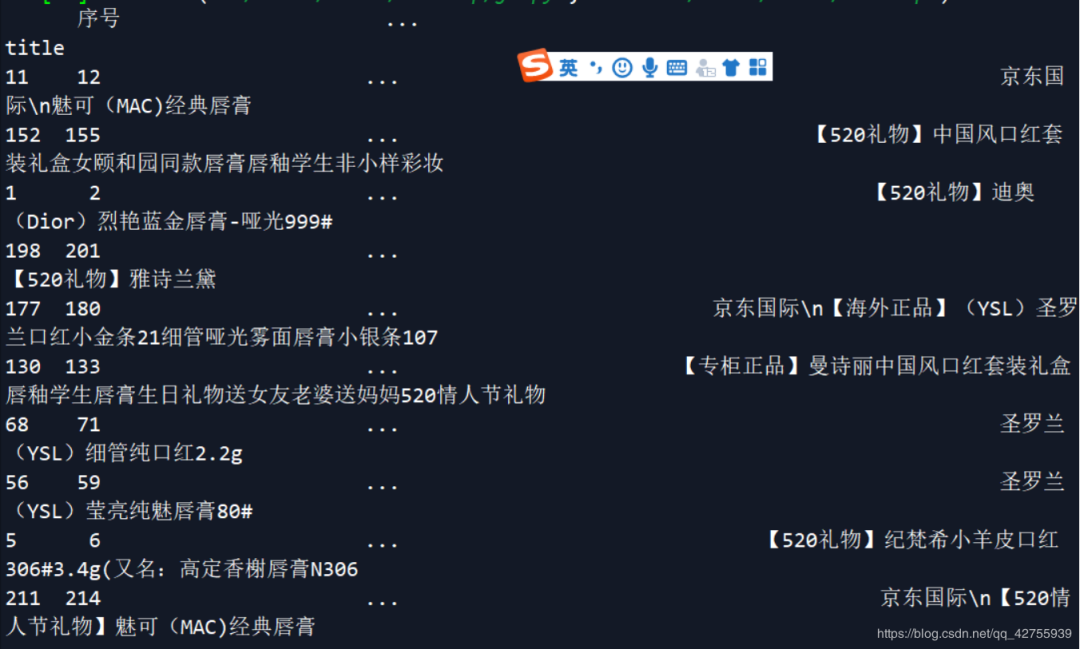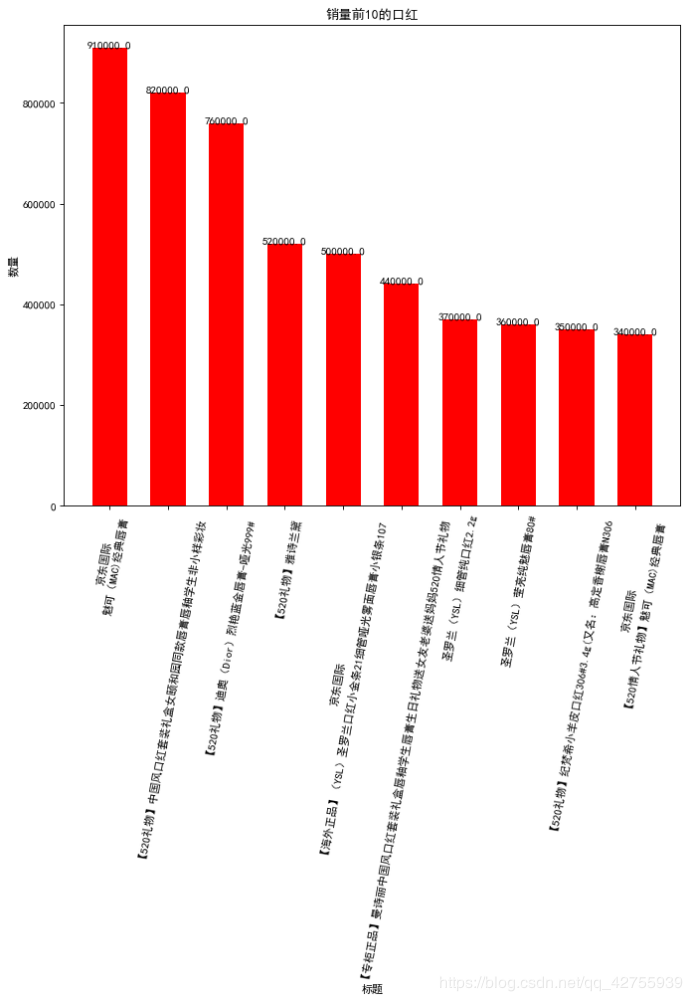1、京东国际魅可（MAC)经典唇膏 子弹头口红3g Chili 小辣椒色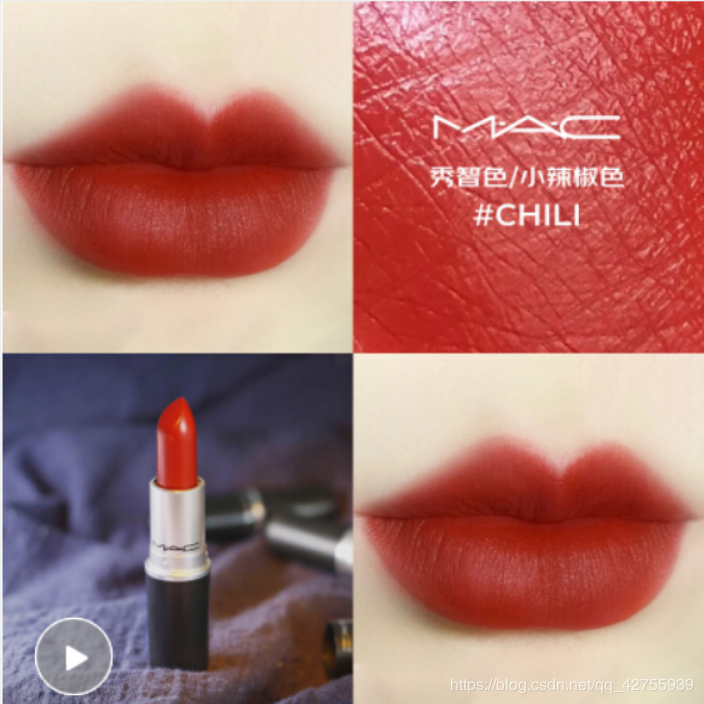2、【520礼物】中国风口红套装礼盒女颐和园同款唇膏唇釉学生非小样彩妆 口红套装（6支）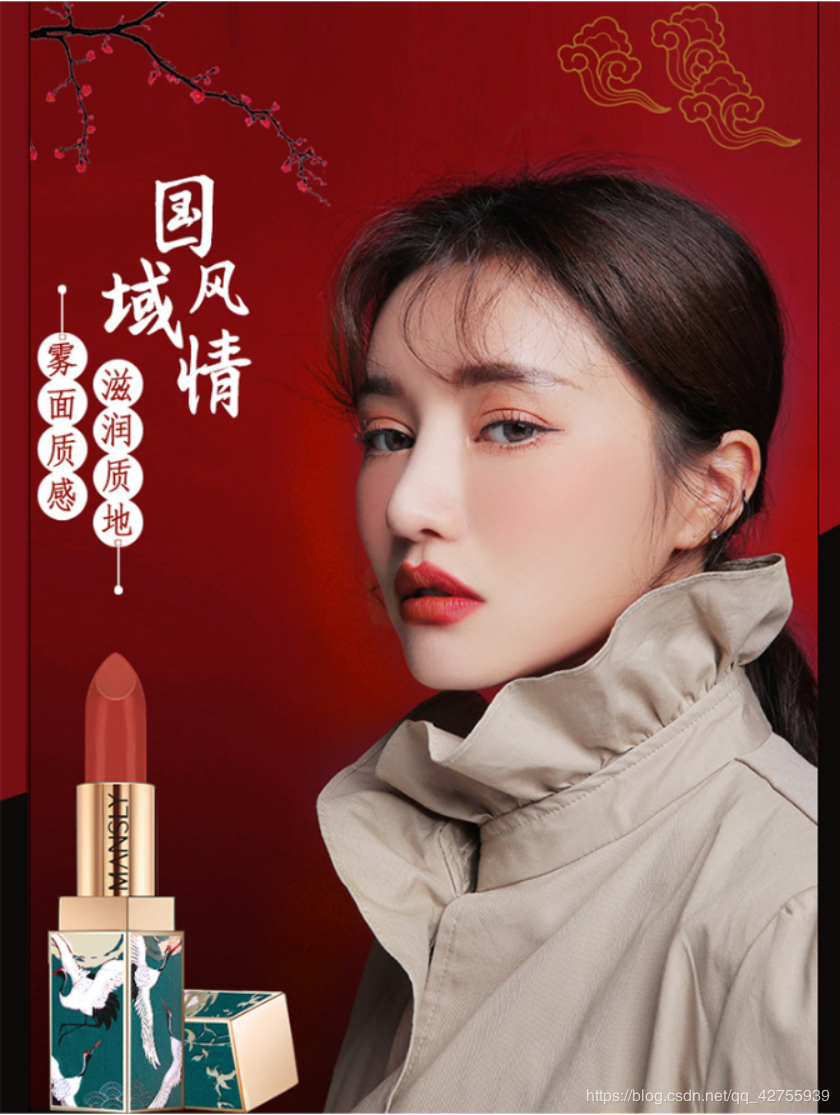3、【520礼物】迪奥（Dior）烈艳蓝金唇膏-哑光999# 3.5g 传奇红（口红 正红色 传奇红 赠精美礼盒）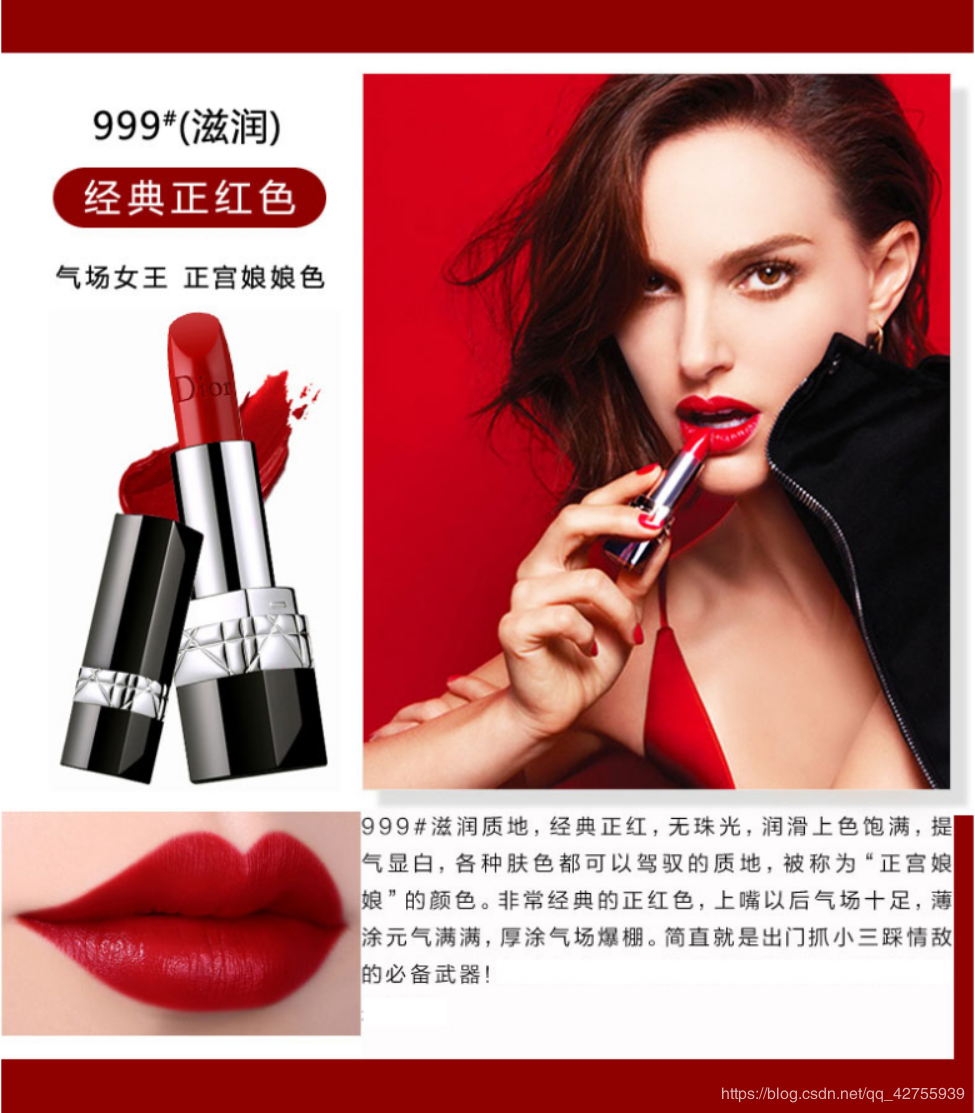#### 4、销量前10的店铺

#销量前10的店铺top_shop = data.groupby('shop_name')['comment'].sum().sort_values(ascending=False)[:10]print(top_shop.head(10))plt.figure(figsize=(10,8),dpi = 80)top_shop.plot(kind = 'bar',color='red',width= 0.6)plt.ylabel('数量')plt.xlabel('店铺名')  plt.title('销量前10的店铺') plt.xticks(rotation=45)for x,y in enumerate(list(top_shop)):     plt.text(x,float(y)+0.1,y,ha='center')plt.show()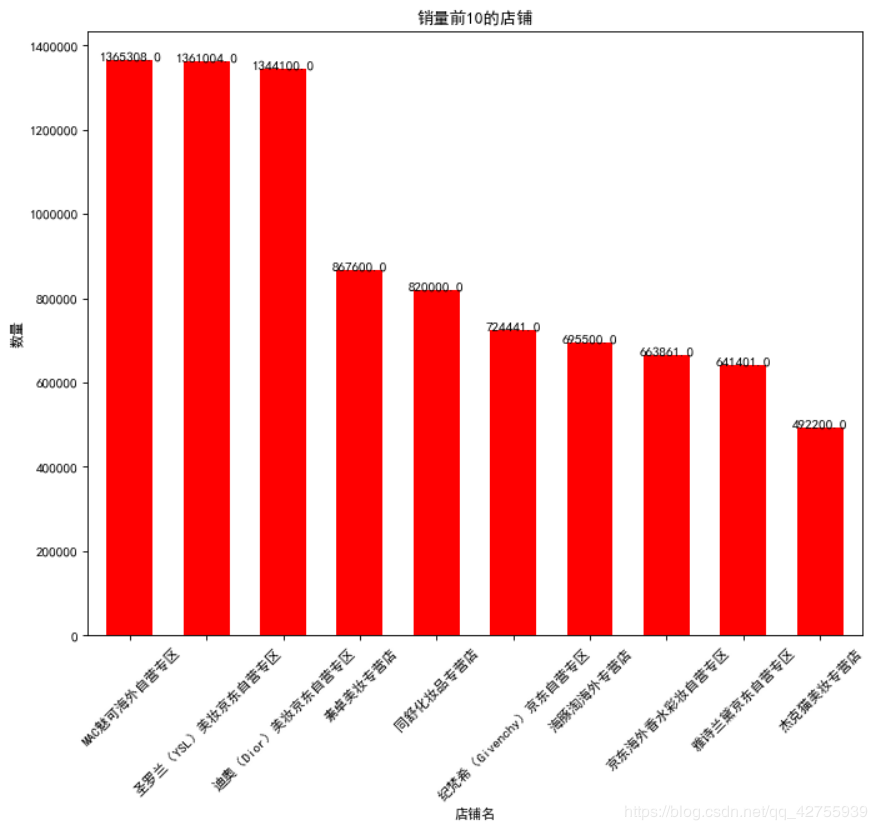1、MAC魅可海外自营专区 占据第一名，达 1365308的销售量，而且基本前10的店铺销量都在5万以上。

2、前三名都基本达到了130多万

3、前10名中有5个是京东自营

### 5、商品价格和销量的关系

plt.figure(figsize=(10,8))plt.scatter(data['price'],data['comment'], color='blue')plt.xlabel('价格')plt.ylabel('销量')plt.title('价格、销量的散点分布')plt.show()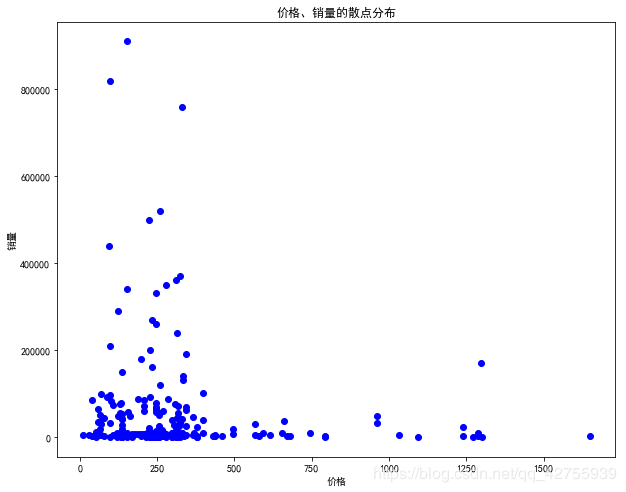### 四、总结

• 数据清洗，它是能分析出正确结果的保证；

• 如何挖掘数据不同维度间的联系等；

• 比如分析不同类型的店铺占比店铺；

• 不同类型的店铺之间的销量对比；

• 由于本次没有爬取评论数据，没有做情感分析；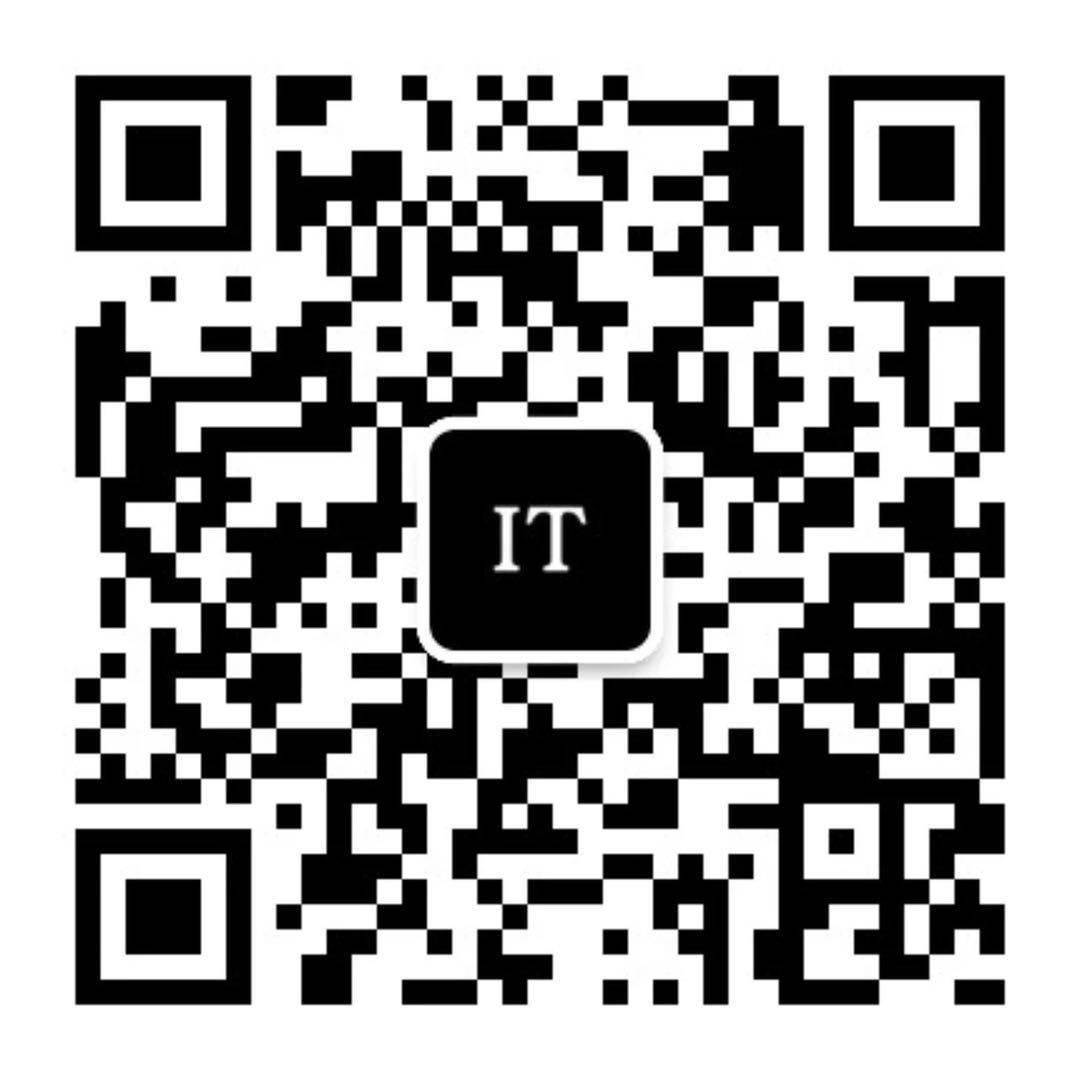## 评论 (3 条评论)2020 年 05 月 30 日 14:21

2020 年 05 月 30 日 14:372020 年 05 月 30 日 13:05

2020 年 05 月 30 日 13:07

2020 年 05 月 30 日 18:33

2020 年 05 月 30 日 21:20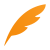2020 年 05 月 29 日 10:01

2020 年 05 月 29 日 15:33